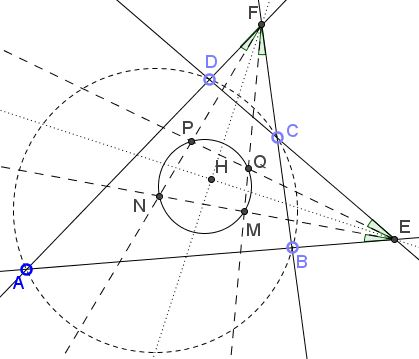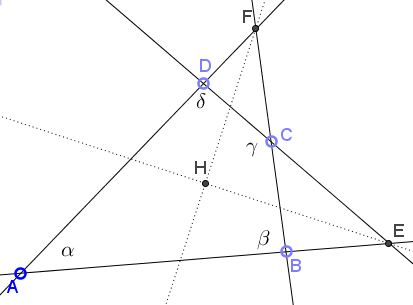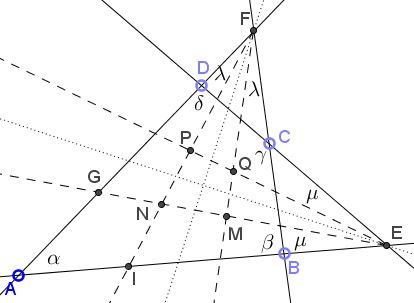# A Family of Cyclic Quadrilaterals

### Problem

Let $ABCD$ be a cyclic quadrilateral; $E$ the intersection $AB\cap CD,$ $F=BC\cap AD.$Prove that

1. The bisectors of angles AFB and AED are orthogonal.

2. Pairs of the isogonal conjugates at each of the angles form a cyclic quadrilateral.

### Solution

The problem is solved by routine "angle chasing." Denote the angles as shown below:For part 1, if $H$ is the intersection of the angle bisectors, then in the (concave) quadrilateral $CEHF,$ the internal angles are found to be

\begin{align} \mbox{at} E: & 90^{\circ}-\displaystyle\frac{\alpha +\delta}{2}\\ \mbox{at} F: & 90^{\circ}-\displaystyle\frac{\alpha +\beta}{2}\\ \mbox{at} C: & 360^{\circ}-\gamma. \end{align}

It follows that,

\begin{align} \angle EHF &=360^{\circ}-\bigg[90^{\circ}-\displaystyle\frac{\alpha +\delta}{2}\bigg]-\bigg[90^{\circ}-\displaystyle\frac{\alpha +\beta}{2}\bigg]-\bigg[360^{\circ}-\gamma\bigg]\\ &=\alpha+\gamma+\displaystyle\frac{\beta +\delta}{2}-180^{\circ}\\ &=180^{\circ}+90^{\circ}-180^{\circ}=90^{\circ}, \end{align}

because in cyclic quadrilateral $ABCD,$ $\alpha+\gamma=180^{\circ}$ and $\beta+\delta=180^{\circ}.$

For the second part, let $\angle AFP=\angle BFQ=\lambda\space$ and $\angle AEM=\angle CEQ=\mu.$ Introduce $G=AF\cap EM$ and $I=AE\cap FP.$We successively calculate

\begin{align} \angle AGE &= 180^{\circ}-\alpha-\mu\\ \angle AIF &= 180^{\circ}-\alpha-\lambda\\ \angle MNP &= \angle GNI = \alpha +\lambda+ \mu\\ \angle EQF &= 360^{\circ}-(360^{\circ}-\gamma)-\lambda-\mu=\gamma-\lambda-\mu. \end{align}

It follows that $\angle MNP+\angle MQP=\alpha+ \gamma=180^{\circ},$ making quadrilateral $MNPQ$ cyclic.

### Acknowledgment

The problem has been posted by Dao Thanh Oai (Vietnam) at the CutTheKnotMath facebook page.